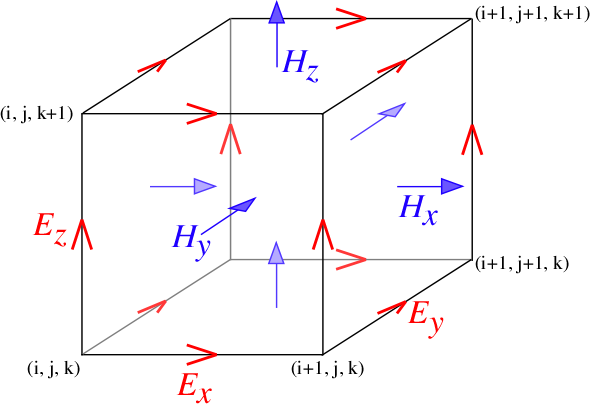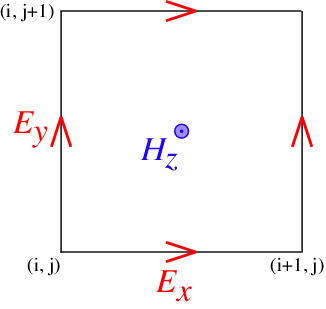# Yee LatticeIn order to discretize Maxwell's equations with second-order accuracy for homogeneous regions where there are no discontinuous material boundaries, FDTD methods store different field components for different grid locations. This discretization is known as a Yee lattice.

The form of the Yee lattice in 3d is shown in the schematic above for a single cubic grid voxel with dimensions $\Delta x \times \Delta x \times \Delta x$. The three components of $\mathbf{E}$ are stored on the edges of the cube in the corresponding directions, while the components of $\mathbf{H}$ are stored on the cube faces.

More precisely, let a coordinate $(i,j,k)$ in the grid correspond to:

where $\hat{\mathbf{e}}_k$ denotes the unit vector in the k-th coordinate direction. Then, the $\ell$th component of $\mathbf{E}$ or $\mathbf{D}$ (or $\mathbf{P}$) is stored for the locations:

The $\ell$th component of $\mathbf{H}$, on the other hand, is stored for the locations:

In two dimensions, the arrangement is similar except that we set $\hat{\mathbf{e}}_3=0$. The 2d Yee lattice for the $H_z$-polarization ($\mathbf{E}$ in the $xy$ plane and $\mathbf{H}$ in the $z$ direction) is shown in the figure below.The consequence of the Yee lattice is that, whenever you need to access field components, e.g. to find the energy density $(\mathbf{E}^* \cdot \mathbf{D} + |\mathbf{H}|^2)/2$ or the flux $\textrm{Re}\, \mathbf{E}^* \times \mathbf{H}$, then the components need to be interpolated to some common point in order to remain second-order accurate. Meep automatically does this interpolation for you wherever necessary — in particular, whenever you compute energy density or Poynting flux, or whenever you output a field to a file, it is stored at the centers of each grid voxel: $(i+0.5,j+0.5,k+0.5)$.

In a Meep simulation, the coordinates of the Yee lattice can be obtained using a field function.